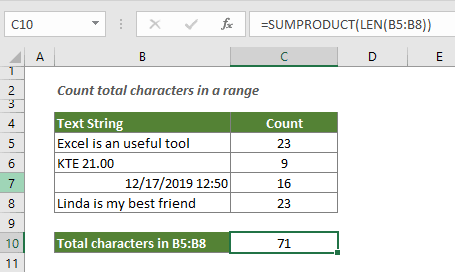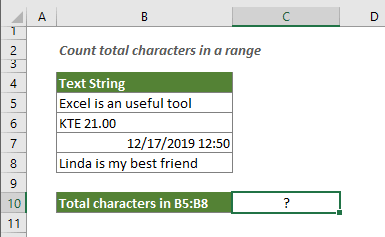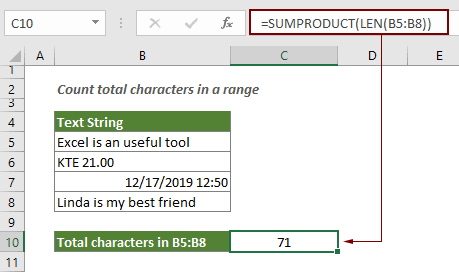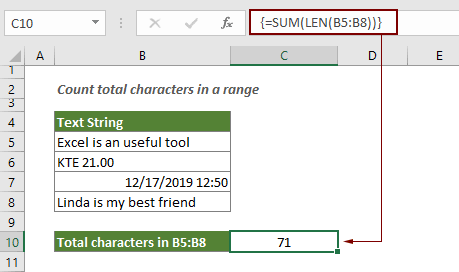Note: The other languages of the website are Google-translated. Back to English

## Count number of characters in a range in Excel

This article explains a formula to count total characters that appear in a range of cells in Excel.#### How to count number of characters in a range of cells in Excel?

Generic formula

=SUMPRODUCT(LEN(range))

Argument

Range: The range for which you want to count the number of all characters.

How to use this formula?

As the below screenshot shown, you want to count all characters in range B5:B8, please do as follows.1. Select a blank cell to output the result.

2. Enter the below formula into it and press the Enter key.

=SUMPRODUCT(LEN(B5:B8))Then you can see all characters including letters, spaces and symbols in specified range are counted.

Tips: Another alternative formula is to combine the SUM function and the LEN function. In this case, enter the below formula into a blank cell, and then press the Ctrl + Shift + Enter keys to get the total characters.
=SUM(LEN(B5:B8))How this formula works

=SUMPRODUCT(LEN(B5:B8))

• 1. LEN(B5:B8): The LEN function calculates the string length for each cell in the specified range, and returns the length as an array of numbers. Here the result is {23;9;16;23};
• 2. SUMPRODUCT({23;9;16;23}): The SUMPRODUCT function sums all numbers in the array and returns the result: 23+9+16+23=71.

#### Related functions

Excel LEN function
The LEN function returns the number of characters in a text string.

Excel SUMPRODUCT function
The SUMPRODUCT function can be used to multiply two or more columns or arrays together, and then get the sum of products.

#### Related formulas

Count occurrences of specific characters in an Excel cell
This tutorial introduces how to apply formulas based on the LEN and SUSTITUTE functions to count the number of times a specific character appears in an Excel cell.

Count specific characters in a range of cells
This tutorial introduces some formulas to count the number of times a specific character appears in a range in Excel.

Count specific words in a cell in Excel

Count specific words in a range in Excel
This article explains a formula to count the number of times a specific word appears in a range of cells in Excel.

Count number of characters in a cell in Excel
The total characters not only includes all letters, but also all spaces, punctuation marks and symbols in the cell. Follow this tutorial to easily count the total characters in a cell with the LEN function in Excel.

### The Best Office Productivity Tools

#### Kutools for Excel - Helps You To Stand Out From Crowd

Would you like to complete your daily work quickly and perfectly? Kutools for Excel brings 300 powerful advanced features (Combine workbooks, sum by color, split cell contents, convert date, and so on...) and save 80% time for you.

• Designed for 1500 work scenarios, helps you solve 80% Excel problems.
• Reduce thousands of keyboard and mouse clicks every day, relieve your tired eyes and hands.
• Become an Excel expert in 3 minutes. No longer need to remember any painful formulas and VBA codes.
• 30-day unlimited free trial. 60-day money back guarantee. Free upgrade and support for 2 years.#### Office Tab - Enable Tabbed Reading and Editing in Microsoft Office (include Excel)

• One second to switch between dozens of open documents!
• Reduce hundreds of mouse clicks for you every day, say goodbye to mouse hand.
• Increases your productivity by 50% when viewing and editing multiple documents.
• Brings Efficient Tabs to Office (include Excel), Just Like Chrome, Firefox, And New Internet Explorer.